# NCERT Solutions (Ex - 4.1, 4.2, 4.3) - Practical Geometry, Maths, Class 8 Notes - Class 8

## Class 8: NCERT Solutions (Ex - 4.1, 4.2, 4.3) - Practical Geometry, Maths, Class 8 Notes - Class 8

The document NCERT Solutions (Ex - 4.1, 4.2, 4.3) - Practical Geometry, Maths, Class 8 Notes - Class 8 is a part of Class 8 category.
All you need of Class 8 at this link: Class 8

Exercise 4.1

Question 1:

AB = 4.5 cm, BC = 5.5 cm, CD = 4 cm, AD = 6 cm, AC = 7 cm

JU = 3.5 cm, UM = 4 cm, MP = 5 cm, PJ = 4.5 cm, PU = 6.5 cm

(iii) Parallelogram MORE

OR = 6 cm, RE = 4.5 cm, EO = 7.5 cm

(iv) Rhombus BEST

BE = 4.5 cm, ET = 6 cm

(i) Given: AB = 4.5 cm, BC = 5.5 cm, CD = 4 cm, AD = 6 cm, AC = 7 cm

Steps of construction:

(a) Draw AB = 4.5 cm.

(b) Draw an arc taking radius 5.5 cm from point B.

(c) Taking radius 7 cm, draw another arc from point A which intersects the first arc at point C.

(d) Join BC and AC.

(e) Draw an arc of radius 6 cm from point A and draw another arc of radius 4 cm from point C which intersects at D.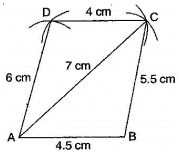(ii) Given:

JU = 3.5 cm, UM = 4 cm, MP = 5 cm, PJ = 4.5 cm, PU = 6.5 cm

Steps of construction:

(a) Draw JU = 3.5 cm.

(b) Draw an arc of radius 4.5 cm taking centre J and then draw another arc of radius 6.5 cm taking U as centre. Both arcs intersect at P.

(c) Join PJ and PU.

(d) Draw arc of radius 5 cm and 4 cm taking P and U as centres respectively, which intersect at M.

(e) Join MP and MU.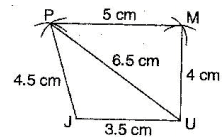(iii) Given: OR = 6 cm, RE = 4.5 cm, EO = 7.5 cm

To construct: A parallelogram MORE.

Steps of construction:

(a) Draw OR = 6 cm.

(b) Draw arcs of radius 7.5 cm and radius 4.5 cm taking O and R as centres respectively, which intersect at E.

(c) Join OE and RE.

(d) Draw an arc of 6 cm radius taking E as centre.

(e) Draw another arc of 4.5 cm radius taking O as centre, which intersects at M.

(f) Join OM and EM.

It is required parallelogram MORE.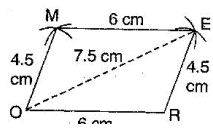(iv) Given: BE = 4.5 cm, ET = 6 cm

To construct: A rhombus BEST.

Steps of construction:

(a) Draw TE = 6 cm and bisect it into two equal parts.

(b) Draw up and down perpendiculars to TE.

(c) Draw two arcs of 4.5 cm taking E and T as centres, which intersect at S.

(d) Again draw two arcs of 4.5 cm taking E and T as centres, which intersects at B.

(e) Join TS, ES, BT and EB.

It is the required rhombus BEST.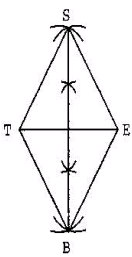Exercise 4.2

Question 1:

LI = 4 cm, IF = 3 cm, TL = 2.5 cm, LF = 4.5 cm, IT = 4 cm

OL = 7.5 cm, GL = 6 cm, GD = 6 cm, LD = 5 cm, OD = 10 cm

(iii) Rhombus BEND

BN = 5.6 cm, DE = 6.5 cm

(i) Given: LI = 4 cm, IF = 3 cm, TL = 2.5 cm, LF = 4.5 cm, IT = 4 cm

Steps of construction:

(a) Draw a line segment LI = 4 cm.

(b) Taking radius 4.5 cm, draw an arc taking L as centre.

(c) Draw an arc of 3 cm taking I as centre which intersects the first arc at F.

(d) Join FI and FL.

(e) Draw another arc of radius 2.5 cm taking L as centre and 4 cm taking I as centre which intersect at T.

(f) Join TF, Tl and TI.

It is the required quadrilateral LIFT.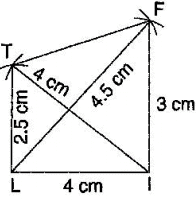(ii) Given:

OL = 7.5 cm, GL = 6 cm, GD = 6 cm, LD = 5 cm, OD = 10 cm

Steps of construction:

(a) Draw a line segment OL = 7.5 cm

(b) Draw an arc of radius 5 cm taking L as centre and another arc of radius 10 cm taking O as centre which intersect the first arc point at D.

(c) Join LD and OD.

(d) Draw an arc of radius 6 cm from D and draw another arc of radius 6 cm taking L as centre, which intersects at G.

(e) Join GD and GO.

It is the required quadrilateral GOLD.(iii) Given: BN = 5.6 cm, DE = 6.5 cm

To construct: A rhombus BEND.

Steps of construction:

(a) Draw DE = 6.5 cm.

(b) Draw perpendicular bisector of line segment DE.

(c) Draw two arcs of radius 2.8 cm from intersection point O, which intersects the line KN at B and N. (d)Join BE, BD as well as ND and NE.

It is the required rhombus BEND.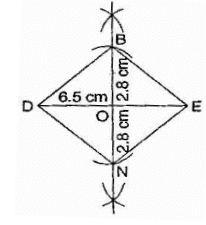Exercise 4.3

Question 1:

MO = 6 cm, OR = 4.5 cm, ∠M = 60% ∠O = 105o , ∠R = 105°

PL = 4 cm, LA = 6.5 cm, ∠P = 90o ∠A = 110o ∠N = 850

(iii) Parallelogram HEAR

HE = 5 cm, EA = 6 cm, ∠R = 850

Rectangle OKAY

OK = 7 cm, KA = 5 cm

(i) Given: MO = 6 cm, OR = 4.5 cm,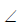M = 60o ,O = 105o ,R = 105o

Steps of construction:

(a) Draw a line segment MO = 6 cm.

(b) ConstructR = 105o and taking radius 4.5 cm, draw an arc taking O as centre, which intersects at R.

(c) Also construct an angle 105o at R and produce the side RE.

(d) Construct another angle of 60o at point M and produce the side ME. Both sides ME and RE intersect at E.

It is the required quadrilateral MORE.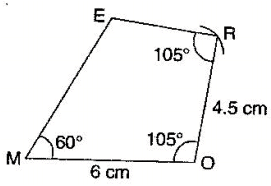(ii) Given: PL = 4 cm, LA = 6.5 cm,P = 90o ,A = 110o ,N = 85o

To find:  ∠L = 3600 - (90 + 85+ 110°) = 360 -285= 75o

Steps of construction:

1. Draw a line segment PL = 4 cm.
2. Construct angle of 90o at P and produce the side PN.
3. Construct angle of 75o at L and with L as centre, draw an arc of radius 6 cm, which intersects at A.
4. Construct ∠A = 110° at A and produce the side AN which intersects PN at N.

It is the required quadrilateral PLAN.(iii) Given: HE = 5 cm, EA = 6 cm,R = 85o

To construct: A parallelogram HEAR.

To find:   ∠H = 180° - 85o = 95°    [ ∵Sum of adjacent angle of ||gm is 1800]

Steps of construction:

1. Draw a line segment HE = 5 cm.
2. Construct ∠H = 95* and draw an arc of radius 6 cm with centre H. It intersects AR at R,
3. Join RH.
4. Draw ∠R = ∠E = 85° and draw an arc of radius 6 cm with E as a centre which intersects RA at A.
5. Join RA

It is the required parallelogram HEAR.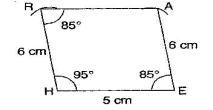(iv) Given: OK = 7 cm, KA = 5 cm

To construct: A rectangle OKAY.

Steps of construction:

1. Draw a line segment OK = 7 cm.
2. Construct angle 90° at both points 0 and K and produce these sides.
3. Draw two arcs of radius 5 cm from points 0 and K respectively. These arcs intersect at Y and A
4. Join YA.

It is the required rectangle OKAY.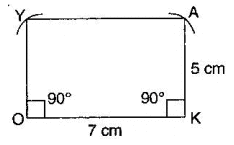The document NCERT Solutions (Ex - 4.1, 4.2, 4.3) - Practical Geometry, Maths, Class 8 Notes - Class 8 is a part of Class 8 category.
All you need of Class 8 at this link: Class 8Use Code STAYHOME200 and get INR 200 additional OFF

Track your progress, build streaks, highlight & save important lessons and more!

,

,

,

,

,

,

,

,

,

,

,

,

,

,

,

,

,

,

,

,

,

,

,

,

,

,

,

,

,

,

,

,

,

;